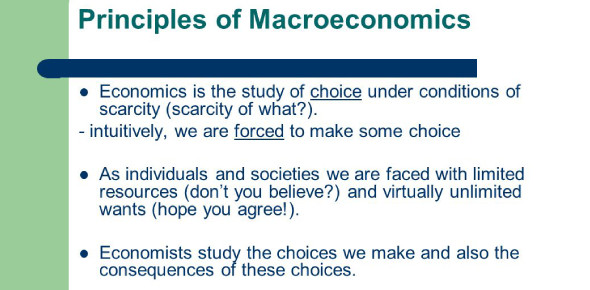# Economics Quiz: Principles Of Macroeconomics! Trivia

19 Questions | Total Attempts: 435SettingsEver wondered how well do you know the principles of macroeconomics? Macroeconomics is a division of finances that deals with implementing, building, behavior, and decision-making of an economy. It utilizes interest rates, taxes, and government spending to manage an economy’s growth and stability. Macroeconomics studies GDP, unemployment rate, national income, investments, savings, and international trade and finance generally deals with large-scale economic factors. This quiz shows you what macroeconomics entails. All the best.

• 1.
Which of the following is a capital resource?
• A.

A new car bought by the Jones family

• B.

A truck used in transporting school children to a soccer practice

• C.

A truck used in transporting steel to an automobile factory

• D.

Hamburger meat used to produce a juicy hamburger on a home grill

• E.

A sapling used to create a forest in a new national park

• 2.
The French production possibilities curve shifts to the right when there is
• A.

A decrease in the French capital stock

• B.

A decrease in the French labor supply

• C.

High unemployment in France during the previous period

• D.

Only consumer goods production in France during the previous period

• E.

Technological innovation in the production of French goods

• 3.
A point inside a production possibilities curve reflects
• A.

The law of increasing costs

• B.

Technological information

• C.

Less than full use of resoures and technology

• D.

Economic efficiency

• E.

A way to increase future economic growth

• 4.
A demand curve shows the relationship between
• A.

Price and quantity demanded

• B.

The demand and supply schedules

• C.

Demand and supply equilibrium

• D.

Leakages and injections

• E.

Price and technology

• 5.
An increase in the demand for a good refers to a shift in the
• A.

Demand curve to the left

• B.

Demand curve to the right

• C.

Quantity demanded to the right

• D.

Quantity demanded to the left

• E.

Price of the good

• 6.
Sugar and honey are substitute goods for many cooking applications. If the price of sugar rises, we would expect the
• A.

Demand for honey to increase

• B.

Demand for honey to decrease

• C.

Quantity demanded of honey to decrease

• D.

Price of honey to decrease

• E.

Quantity demanded of honey to increase

• 7.
A market-day supply curve is
• A.

Horizontal, summing individual supply curves

• B.

Vertical

• C.

Downward sloping

• D.

Upward sloping

• E.

Dependent on the market-day demand

• 8.
If the price of a good is below its equilibrium level, then
• A.

An excess supply will result

• B.

Quantity supplied exceeds quantity demanded

• C.

The supply curve will shift to the right

• D.

Quantity demanded exceeds quantity supplied

• E.

The demand curve will shift to the left

• 9.
If more people enter medical school, we can expect
• A.

The demand for doctors to increase

• B.

The supply of doctors to increase

• C.

The demand for doctors to decrease

• D.

The supply of doctors to decrease

• E.

No effect on the supply or demand of doctors--just a movement along the curves

• 10.
The simultaneous occurrence of inflation and unemployment is called
• A.

Depression

• B.

Downturn

• C.

Deflation

• D.

Demand-pull inflation

• E.

Stagflation

• 11.
If our government cuts taxes, then we will see
• A.

An upward movement along the AD curve

• B.

A downward movement along the AD curve

• C.

The AD curve shift to the left

• D.

The AD curve shift to the right

• E.

The AS curve shift to the right

• 12.
Real GDP is nominal GDP adjusted for
• A.

Price changes

• B.

Intermediate goods

• C.

• D.

• E.

Depreciation

• 13.
The expenditure approach to GDP accounting includes
• A.

Wages and salaries

• B.

Net exports

• C.

Net interest

• D.

Corporate profit

• E.

Proprietors' income

• 14.
When net exports are positive,
• A.

Exports are greater than imports

• B.

Imports are greater than exports

• C.

Imports are greater than investments

• D.

Exports are greater than depreciation spending

• E.

Exports are greater than net investment

• 15.
The "life cycle" hypothesis states that
• A.

MPC remains constant as national income rises

• B.

MPC varies with age

• C.

Transitory income determines consumption

• D.

MPC varies with permanent income

• E.

Consumption spending is independent of income

• 16.
Economists refer to the simple relationship between consumption and income as
• A.

Autonomous consumption

• B.

The marginal propensity to consume

• C.

The absolute income hypothesis

• D.

Disposable income

• E.

The consumption function

• 17.
The short run consumption curve may shift upward over the long run largely because
• A.

People become wealthier

• B.

The marginal propensity to save increases

• C.

The 45-degree line becomes a 90-degree line

• D.

The demand curve for investment is upward sloping

• E.

Economic growth, although still positive, slows down

• 18.
The relationship between MPC and MPS is
• A.

1+MPC=MPS

• B.

1-MPC=MPS

• C.

1+MPS=MPC

• D.

MPC-MPS=1

• E.

MPS-MPC=1

• 19.
When the interest rate falls, the level of investment in the economy
• A.

Falls

• B.

Is unaffected

• C.

Shifts to the left

• D.

Rises

• E.

Shifts to the right

Related TopicsBack to top